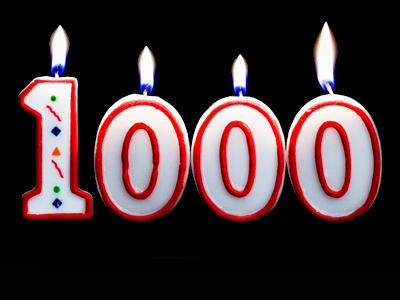What is 4,501 rounded to the nearest 1,000?

# Rounding Numbers (Easy)

When you round a number, you write it as an approximation of the original number: numbers can be rounded upwards and downwards. For example, 127 rounded to the nearest ten is 130 because 27 is closer to 30 than to 20. If you round 127 to the nearest hundred, it becomes 100 because 127 is nearer to 100 than to 200. All is well until someone asks you to round a number like 125 to the nearest ten: 25 is equidistant (the same distance) from 20 and 30. What do you do in such cases? You follow this RULE:

If the number that you use to decide whether to round upwards or downwards (let's call it the deciding number) is halfway or closer to the number to which you have to round to, round upwards BUT if it is less than halfway to the number to which you have to round to, round downwards.

Try these 11-plus Maths questions and see how easy rounding really is.

1.
What is 2,389 rounded to the nearest 1,000?
2,000
3,000
2,400
2,300
2,389 is closer to 2,000 than to 3,000, so you round downwards
2.
What is 16 rounded to the nearest 10?
10
20
15
30
16 is closer to 20 than 10, so you round upwards
3.
What is 745 rounded to the nearest 10?
700
740
750
700
Although 45 is equidistant from 50 and 40, the rule means that you round upwards
4.
What is 12,576 rounded to the nearest 1,000?
13,000
12,000
13,600
12,600
12,576 is closer to 13,000 than 12,000, so you round upwards
5.
What is 23,452 rounded to the nearest 100?
23,450
23,400
23,000
23,500
452 is closer to 500 than to 400, so you round upwards
6.
What is 675 rounded to the nearest 10?
670
600
680
700
Although 75 is equidistant from 80 and 70, the rule means that you round upwards
7.
What is 687 rounded to the nearest 100?
600
700
680
500
687 is closer to 700 than to 600, so you round upwards
8.
What is 3,335 rounded to the nearest 100?
3,400
3,300
3,000
3,200
335 is closer to 300 than to 400, so you round downwards
9.
What is 4,501 rounded to the nearest 1,000?
5,000
4,000
4,500
5,500
4,501 is closer to 5,000 than to 4,000, so you round upwards
10.
What is 10,007 rounded to the nearest 10?
10,000
10,010
10,100
10,110
7 is closer to 10 than to 0, so you round upwards
Author:  Frank Evans

We use cookies to make your experience of our website better.

To comply with the new e-Privacy directive, we need to ask for your consent -